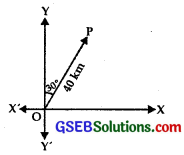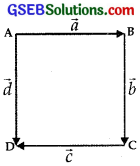# GSEB Solutions Class 12 Maths Chapter 10 Vector Algebra Ex 10.1

Gujarat Board GSEB Textbook Solutions Class 12 Maths Chapter 10 Vector Algebra Ex 10.1 Textbook Questions and Answers.

## Gujarat Board Textbook Solutions Class 12 Maths Chapter 10 Vector Algebra Ex 10.1

Question 1.
Represent graphically a displacement of 40 km, 30° east of north.
Solution:
A line segment of 2 cm is drawn on the right of OY making an angle of 30° with it.
OP = 40 km, scale 1 cm = 20 km.
Vector $$\overline{\mathrm{OP}}$$ represents displacement of 40 km, 30° east of north.Question 2.
Classify the following measures as scalars and vectors:
(i) 10 kg
(ii) 2 metres north-west
(iii) 40°
(iv) 40 watt
(v) 10-10 columb
(vi) 20 m/sec2
Solution:
Scalars:
(i) 10 kg
(iii) 40°
(iv) 40 watt
(v) 10-10 columb

Vectors:
(ii) 2 metres north-west
(vi) 20 m/sec2Question 3.
Classify the following as scalar and vector quantities:
(i) time period
(ii) distance
(iii) force
(iv) velocity
(v) work
Solution:
Scalar Quantity:
(i) time
(ii) distance
(v) work

Vector Quantity:
(iii) force
(iv) velocityQuestion 4.
In a square, identify the following vectors:
(i) co-initial
(ii) equal
(iii) collinear but not equal
Solution:
(i) Coinitial vectors are $$\vec{a}$$ and $$\vec{d}$$.
(ii) Equal vectors are $$\vec{b}$$ and $$\vec{d}$$.
(iii) Collinear but not equal vectors are $$\vec{a}$$ and $$\vec{c}$$.Question 5.
Answer the following as true and false:
(i) $$\vec{a}$$ and – $$\vec{a}$$ are collinear.
(ii) Two collinear vectors are always equal in magnitude.
(iii) Two vectors having the same magnitude are collinear.
(iv) Two collinear vectors having same magnitude are equal.
Solution:
(i) True
(ii) False
(iii) False
(iv) False.